### Week 6: Fractal Reflections; Room Simulation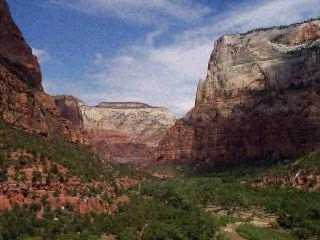Zion Canyon, Utah

#### Fractal Reflections

Last week we discussed the use of fractal techniques to generate various parameters of music. In this class, we took a slightly different approach to using fractals than the "typical" mapping onto pitch/frequency or rhythm, etc. (it's amazing how many people think of algorithmic composition as working only in these two domains). Fractal geometry is a very powerful way to represent natural forms -- in fact, much of Mandelbrot's original investigations into fractals dealt with issues of representing these forms; the coastline of Britain, the size (area) of clouds, etc. His book is titled The Fractal Geometry of Nature, after all!

As an example, the following image was generated entirely by fractal techniques:[NOTE: If you would like to experiment with creating your own fractal landsscapes, the xmountains package (complete with source code!) is a good place to start. We showed this in class.]

The ability to capture essential features of landscapes leads to interesting possibilities. One of my favorite sounds in the world is the echoes and reflections that come from mountain and canyon walls. Last summer, I stood for some time yelling things like "Hey!" and clapping my hands while facing the Zion Canyon walls shown in the picture at the top of the page. Lacking the financial resources or time to conveniently travel to Utah whenever I would like to re-create this sound, I of course turn to the computer for assistance. We can use fractal techniques to simulate this sound.

It is important to realize that an echo is basically nothing more than a copy of a sound played at a later time. We already have the ability to do this (play a sound at some future time) using the RTcmix STEREO instrument. All we need to do is generate the correct time-point data to duplicate the reflections from a fractal boundary.

To do this, we need to write a program that will do this. The essence of creating a fractal is the notion of recursion, or applying the same operation over and over again -- but supplying new data points (generated from the previous data points) at each level of the recursion. For example, one of the most famous fractals is a thing called the Koch Snowflake Curve. It is created by taking a basic shape: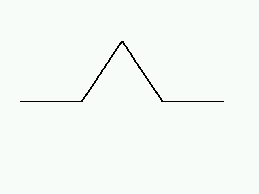and then applying the procedure used to make the basic shape to each sub-line of the basic shape (recursion level 1):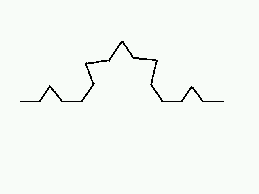and then doing this again (recursion level 2):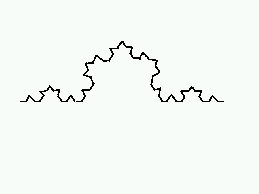and again and again... etc. Now suppose we came up with a procedure to fractally generate a canyon-like wall, looking something like this: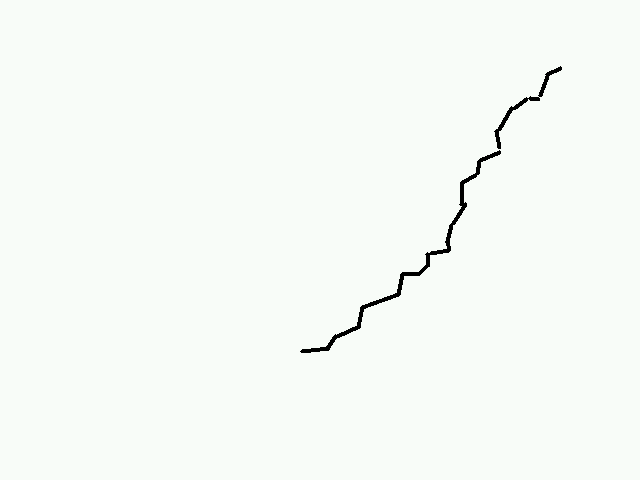If we posit a hypothetical person standing a distance away from the canyon, perhaps yelling "hey!", we can imagine that the sound will travel from the source towards the virtual canyon wall: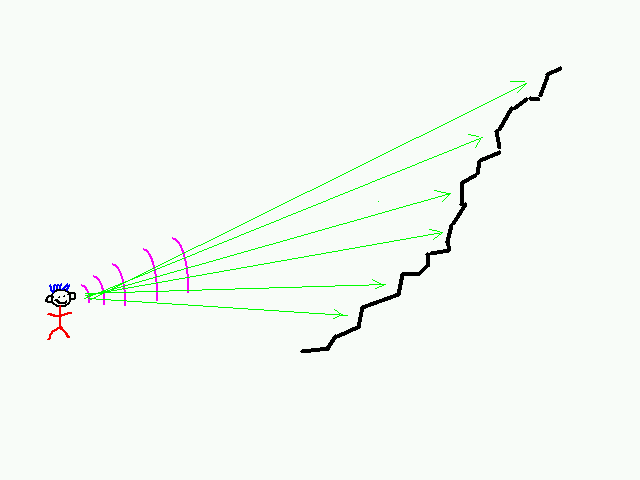The sound waves would reflect from the canyon wall back towards the person, each one delayed by the distance travelled to reach a particular reflecting face of the wall: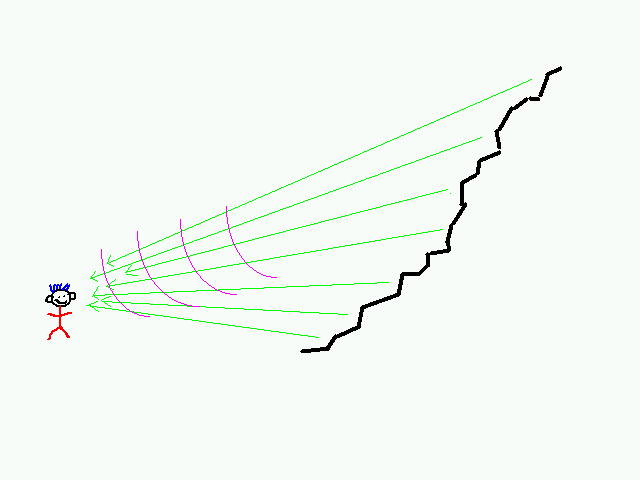Now assuming that sound travels at about 1100 feet/second, we are going to make several assumptions about our sonic model. Instead of calculating the angles and exact path-lengths of every reflection, we will map the fractal canyon wall down to a set of delay points along the ground, essentially mapping the canyon wall from 2 dimensions to 1 dimension:The delay points would thus be generated by the (red) intersections within the (green) time span below: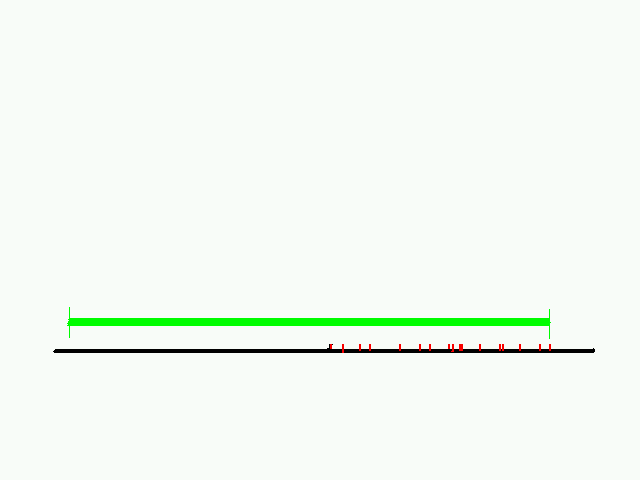If we assume that the farthest point on the canyon wall is slightly less than 550 feet from our source/listener person, and that the canyon wall starts at some arbitrary distance from the source/listener person less than this, then all we have to do is come up with a recursive technique for dividing up points of time between 0 and 0.5 seconds. Each point we generate will become a single delay, or a single call to STEREO with a particular output skip parameter that will generate that delay.

Although this sounds complicated, it is actually rather easy to do. Suppose that we decide to generate fractal faces of our canyon wall by deciding that each segment will have a face-point (sound reflection point) at 70% of the "base" face. Calculating the time delays is easy:

1. Take the time span 0.0 -- 0.5, multiply it by 0.7 to get the first delay point (0.5 * 0.7 = 0.35).

2. Take the "cut" time spans (0.0 -- 0.35; 0.35 -- 0.5) and multiply each one of these by 0.7 to get the second set of time delay points (0.35 * 0.7 = 0.245; (((0.5-035) * 0.7) + 0.35 = 0.455). [This is recursion level 1]

3. Take each of those "cut" time spans ( 0.0 -- 0.245; 0.245 -- 0.35; 0.35 -- 0.455; 0.455 -- 0.5) and apply the same procedure...[This is recursion level 2]

4. etc.

Here's a crude graphical depiction of the above: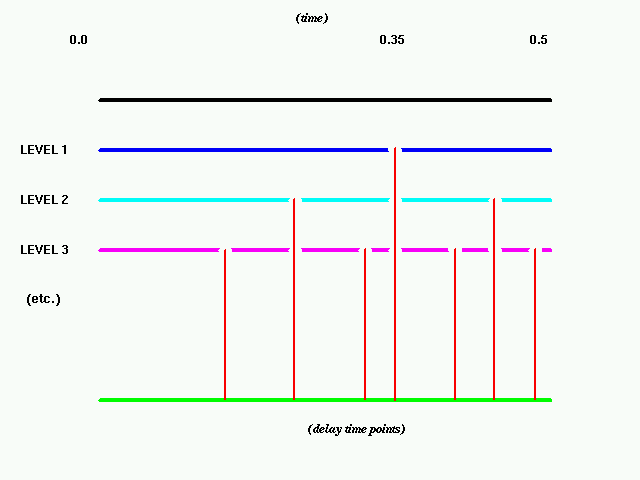The code to do this is not complicated at all. Again, the main concept to get around is the use of recursion in this procedure -- note that the gendelays() function calls itself twice, with different pieces of the "cuts" each time. We also check the level (in the following code it is set at "4") to be sure that we don't continue cutting into smaller and smaller pieces forever(level is passed as one of the parameters for gendelays()).

The code (compile with a simple "cc"):

```#include <stdio.h>

main()
{
void gendelays(float, float, int);
float start, end;
int level;

start = 0.0;
end = 0.5;
level = 0;

gendelays(start, end, level);
}

void gendelays(float st, float end, int level)
{
float mid;

mid = ((end-st) * 0.7) + st;

printf("STEREO(%f, 0.0, 3.0, 0.1, 0.5)\n", mid);

level = level+1;
if (level > 4) return;

gendelays(st, mid, level);
gendelays(mid, end, level);
}
```
If we collect the output of this program into a file, add the appropriate rtsetparams() and rtinput() lines, we can run the resulting scorefile and hear the reflections from the fractal wall!

As a matter of fact, you can hear them right now!:

Hey! -- recording of original soundfile (brad yelling "hey!")
fractal1 -- fractal-delayed version with number of recursion levels set to 4
fractal2 -- fractal-delayed version with number of recursion levels set to 7
Although this simulation of the reflection delay patterns is surprisingly good, there is a regularity to it that signals "artificiality" to the ear (believe it or not, this becomes more pronounced the more levels of recursion there are (why?)). No natural form will ever have an exact fractal dividing of 0.7 for every face -- instead the recursive procedure slightly varies from one level to the next. We can imitate this by adding a slight amount of randomness to the time-points we generate, and using this randomness prior to subsequent recursive calls so that it will be reflected in the overall fractal structure that we generate. The change is very minor -- we take the orginal version of the gendelays() function:
```void gendelays(float st, float end, int level)
{
float mid;

mid = ((end-st) * 0.7) + st;

printf("STEREO(%f, 0.0, 3.0, 0.1, 0.5)\n", mid);

level = level+1;
if (level > 4) return;

gendelays(st, mid, level);
gendelays(mid, end, level);
}
```
and add one additional line of code, using the rrand() function (returns a value between -1.0 and +1.0):
```void gendelays(float st, float end, int level)
{
float mid;

mid = ((end-st) * 0.7) + st;
mid = mid + (rrand() * 0.01);

printf("STEREO(%f, 0.0, 3.0, 0.1, 0.5)\n", mid);

level = level+1;
if (level > 4) return;

gendelays(st, mid, level);
gendelays(mid, end, level);
}
```
Of course, because we've done this we will need to #include the following line at the top of the file:
```#include "/musr/cmix/H/randfuncs.h"
```
and compile it by saying: "cc -o fract2 fract2.c /musr/cmix/lib/randfuncs.o -lm" (assuming of course that the file containing the code is "fract2.c").

The results of this version can be heard with this mp3 file:

randomized fractal -- soundfile generated using slight amounts of randomness in the fractal procedure

A CSOUND Instrument

coming soon...

#### Room Simulation

Calculating reflections from a fractal boundary is very similar to approaches used in simulating different room acoustics. Basically, many of these approaches use a technique analogous to "ray tracing" in creating models of light used for graphics. The trick is to find the optimum reflection from th walls in a virtual room you are modelling -- optimum being where the angle of incidence is equal to the angle of reflection -- and to use the distance to dictate delay poin.

More coming later...

Assignments:

1. Account for amplitude in the calculation of the delays (hint: the amplitude of sound is inversely proportional to the square of the distance travelled).

2. Use fractal techniques to control some other parameter of sound, perhaps timbre-construction.

3. Account for three-dimenional "spatialization"; include several different surfaces (and possibly reflective objects) (hint: include the timing differences between ears of the listener).